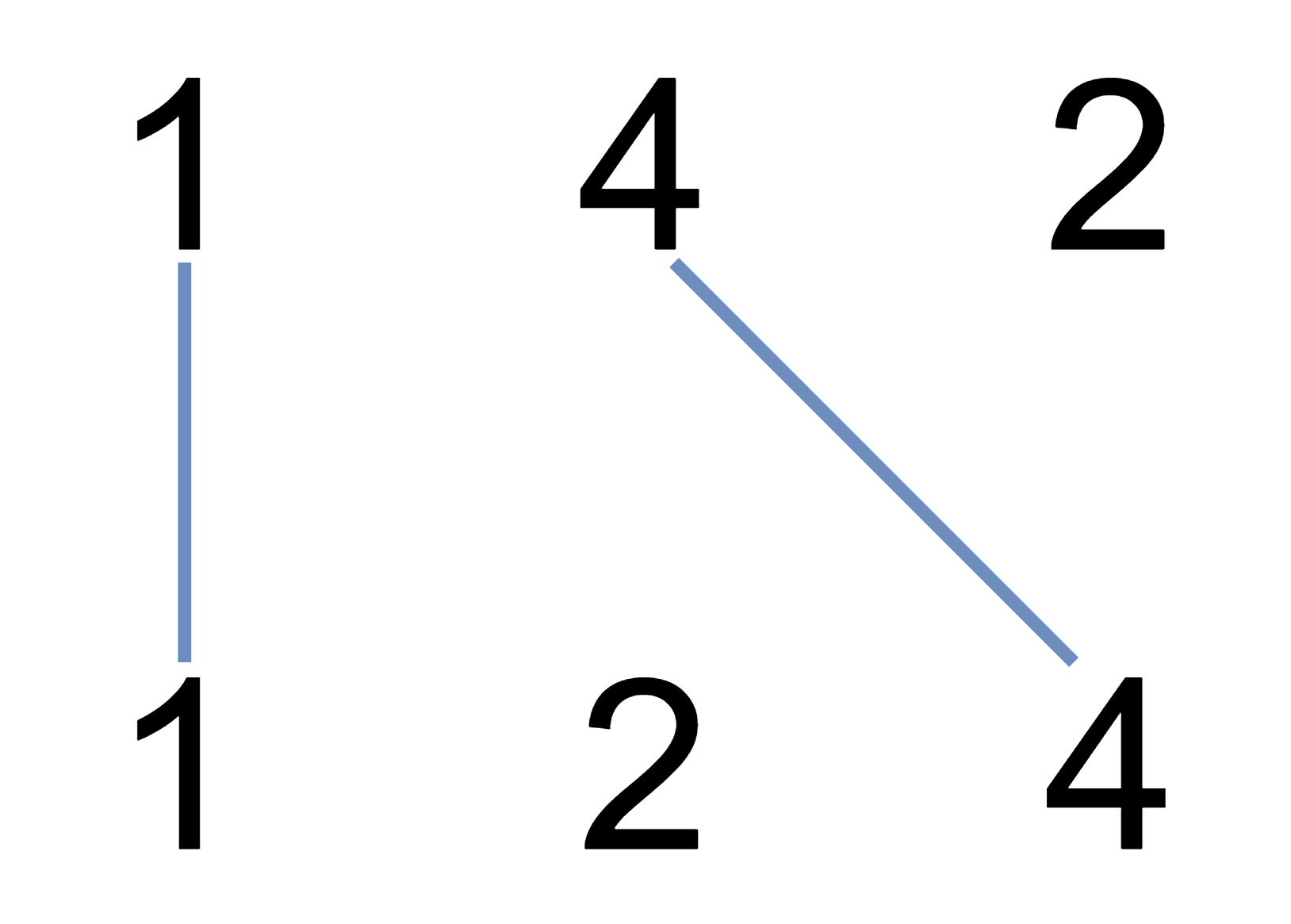1035. Uncrossed Lines
Medium
3.5K
44

You are given two integer arrays `nums1` and `nums2`. We write the integers of `nums1` and `nums2` (in the order they are given) on two separate horizontal lines.

We may draw connecting lines: a straight line connecting two numbers `nums1[i]` and `nums2[j]` such that:

• `nums1[i] == nums2[j]`, and
• the line we draw does not intersect any other connecting (non-horizontal) line.

Note that a connecting line cannot intersect even at the endpoints (i.e., each number can only belong to one connecting line).

Return the maximum number of connecting lines we can draw in this way.

Example 1:```Input: nums1 = [1,4,2], nums2 = [1,2,4]
Output: 2
Explanation: We can draw 2 uncrossed lines as in the diagram.
We cannot draw 3 uncrossed lines, because the line from nums1 = 4 to nums2 = 4 will intersect the line from nums1=2 to nums2=2.
```

Example 2:

```Input: nums1 = [2,5,1,2,5], nums2 = [10,5,2,1,5,2]
Output: 3
```

Example 3:

```Input: nums1 = [1,3,7,1,7,5], nums2 = [1,9,2,5,1]
Output: 2
```

Constraints:

• `1 <= nums1.length, nums2.length <= 500`
• `1 <= nums1[i], nums2[j] <= 2000`
Accepted
134.1K
Submissions
216.5K
Acceptance Rate
61.9%

Seen this question in a real interview before?
1/4
Yes
No

Discussion (0)

Related Topics